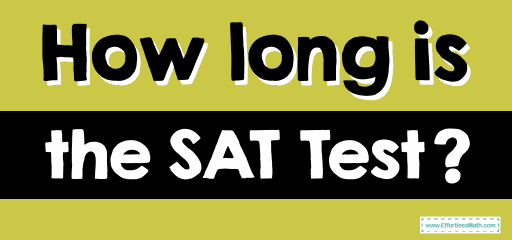# How long is the SAT Test?To some students, the SAT may seem like it lasts forever, but it is a timed test. The SAT is generally three hours (180 minutes), with no optional essay and rest. With the optional essay, the total test time is increased to three hours and 50 minutes. The timing of each section is as follows:

## The Absolute Best Book to Ace the SAT Math Test

• Reading: 65 minute- 52 questions (75 seconds per question)
• Writing and Language: 35 minute- 44 questions (about 47 seconds per question)
• Math – No-Calculator: 25 minute- 20 questions (75 seconds per question)
• Math – Calculator: 55 minute-38 questions (about 87 seconds per question)
• Essay (optional): 50 minutes, one essay

The math section is the only section that is divided into two sections: No- Calculator and a Calculator section. As you can see, the No-Calculator section is first and asks 20 questions in 25 minutes, while the calculator section is in second place and asks 38 questions in 55 minutes. In total, you will have 58 questions and 80 minutes for math.

You will also have a total of three breaks (if you do not use the optional essay, twice):

• 10 minutes break after Reading but before Writing and Language
• A five-minute rest after the Math No-Calculator section but before the Math Calculator section
• A very short break of two minutes after the Math Calculator section (if you stay in the Essay section, which means otherwise you are done and may leave!)

## College Entrance Tests

Looking for the best resource to help you succeed on the SAT Math test?

## The Best Books to Ace the SAT Math Test

### What people say about "How long is the SAT Test? - Effortless Math: We Help Students Learn to LOVE Mathematics"?

No one replied yet.

X
30% OFF

Limited time only!

Save Over 30%

SAVE \$5

It was \$16.99 now it is \$11.99